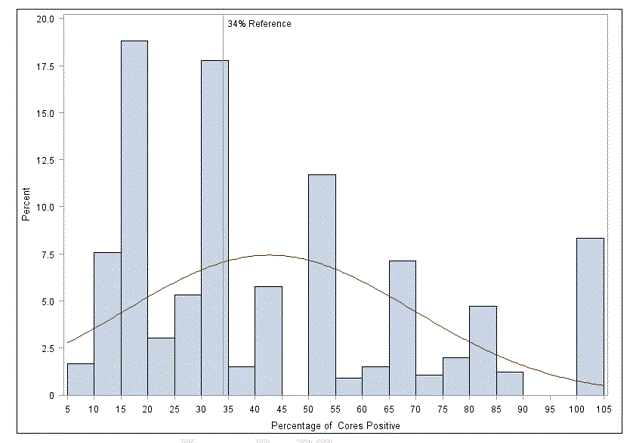## RE: Histogram of percentages (100% max) but showing 105% due to bin of 5

Hi All,

I'm trying to run a proc univariate to get a histogram of percentages of cores positive (please see attached for the data in excel format). Included in the dataset are the percentages, with maximum percentage of 100%. However, when I tried to graph the histogram, I get 105%. I tried to modified the bin but that didn't help. Could any of you please kindly help?

This is my code:

Proc univariate data=cores_positive; var percent_pos_core; histogram percent_pos_core/normal endpoints=(5 to 90) HREF=34 HREFLABELS ="34% Reference"; Run;

Running this code, I got the figure below. I would like the 100% to be at 100%, not 105%Thank you very much!

Tien

1 ACCEPTED SOLUTION

Accepted Solutions

## Re: RE: Histogram of percentages (100% max) but showing 105% due to bin of 5

By default, the bins include the left-hand endpoints

[0, 5), [5, 10), ... [95, 100)

so if there is a 100 value in the data, then the algorithm will add a new interval [100, 105).

To get the bins to include the right-hand endpoint, use the RTINCLUDE option on the HISTOGRAM stmt:

``````data Have;
do i = 1 to 1000;
x = rand("integer", 1, 100);
output;
end;
run;

proc univariate data=Have;
histogram x / endpoints=(0 to 100 by 5) rtinclude;
run;``````

If your data ALSO includes the value 0, then using RTINCLUDE will add a bin at (-5, 0], but it looks like this isn't relevant to your data.

6 REPLIES 6

## Re: RE: Histogram of percentages (100% max) but showing 105% due to bin of 5

By default, the bins include the left-hand endpoints

[0, 5), [5, 10), ... [95, 100)

so if there is a 100 value in the data, then the algorithm will add a new interval [100, 105).

To get the bins to include the right-hand endpoint, use the RTINCLUDE option on the HISTOGRAM stmt:

``````data Have;
do i = 1 to 1000;
x = rand("integer", 1, 100);
output;
end;
run;

proc univariate data=Have;
histogram x / endpoints=(0 to 100 by 5) rtinclude;
run;``````

If your data ALSO includes the value 0, then using RTINCLUDE will add a bin at (-5, 0], but it looks like this isn't relevant to your data.

## Re: RE: Histogram of percentages (100% max) but showing 105% due to bin of 5

Thank you very very much Rick! You are the best!

Sincerely,

Tien

## Re: RE: Histogram of percentages (100% max) but showing 105% due to bin of 5

For more information about setting the bins for histograms (PROC UNIVARIATE and PROC SGPLOT), see "Choosing bins for histograms in SAS."

## Re: RE: Histogram of percentages (100% max) but showing 105% due to bin of 5

Thank you Rick for posting more information on proc univariate and proc sgplot!

Tien